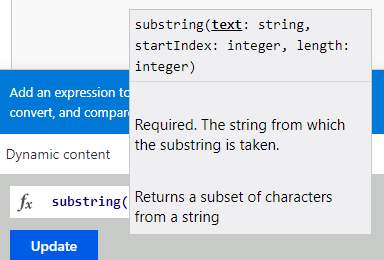# How Substring Works in Microsoft FlowIn most programming languages, we have access to an extremely helpful function called substring. We can use this function to extract snippets of a string that we need the most. Microsoft Flow is no different in the fact that it offers us the use of this function, but the way we use it is a bit different than typical modern approaches.

## How Does Substring Work in Microsoft Flow?

In a programming language like JavaScript, we can use the substring functionality in various ways. Microsoft Flow, however, has one way of using it. When entering the substring expression into a Flow, you will see the inputs required to complete the function (shown below).This looks relatively standard, but the one part of this that might throw you off is the length parameter. In normal conditions, the length of the string would simply be exactly that, the length of the entire string you are trying to pull a substring out of. However, in this case, if we wanted to subtract a file extension from the name of a file, it would not work.

Let’s take MyWordDocument.docx as an example.

We want to extract “docx” from the string. Typically, we could use the string as the “text” parameter (which would be MyWordDocument.docx), start index would be the index in which the period is, and length would be the entire length of the string. If we do this, we will encounter an error saying that the length parameter we used cannot be longer than the length of the string itself.

There’s a reason this happens — the length is assumed from the starting index. If we had a starting index of 5 and the length of the string was 8, we would come out with a length of 13 instead of the original length of 8, resulting in the error.

## How to Use Substring without Error

In order to remedy this problem, we need to do a couple of extra steps. Let’s initialize four parameters in our Microsoft Flow: FileExtension, IndexOfPeriod, LengthOfString, and NewLength as shown below.First, we will get the index of the period in the string using the following expression:

indexOf(“MyWordDocument.docx”,‘.’)

We can store this in the “IndexOfPeriod” variable by using the “Set Variable” command in Flow. Next, we can store the entire length of the string in our “LengthOfString” variable using the following expression:

length(“MyWordDocument.docx”)

Now, in order to get the actual length, we are going to put into the substring function, we need to subtract the full length of the string from the index in which the period sits. We can store this in the “NewLength” variable and process this using the following expression:

sub(variables(‘LengthOfString’),variables(‘IndexOfPeriod’))

Finally, we can use the substring function to store the final output (which should just be “docx”) in our “FileExtension” variable using the following expression:

substring(“MyWordDocument.docx”,variables(‘IndexOfPeriod’),variables(‘NewLength’))

With that, we should now see that the FileExtension variable now stores the output “docx”, which is exactly what we were looking for.  Below is a screenshot of how the Set Variable commands look within Microsoft Flow.If you have any questions about Microsoft Flow, please reach out to our team here!